# Percentage Worksheets for Grade 5Q.1) Convert 4/5 into Percentage.
a) 80 %
b) 50 %
c) 60 %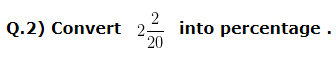a) 230 %
b) 220 %
c) 210 %

Q.3) Convert 75 % into a Fraction and simplify the result to its lowest term .
a) 3/4
b) 5/4
c) 7/4

Q.4) Convert 25 % into decimal .
a) 0.19
b) 0.21
c) 0.25

Q.5) How much Percentage of 6 kg is 300 g .
a) 10 %
b) 5 %
c) 3 %

Q.6) Find 12 % of 25?
a) 3
b) 1
c) 7

Q.7) In a School 70 % of the Students are Girls . What is the percentage of Boys in the School ?
a) 20 %
b) 15 %
c) 30 %

Q.8) In an exam Divya got 30 marks out of 50 . What percentage of marks did she get ?
a) 65 %
b) 60 %
c) 55 %

Q.9) There are 88 children in a class . 75 % of them chose Sanskrit as an extra subject. The rest chose Hindi . How many of them chose Sanskrit and how many chose Hindi ?
a) Number of children who chose Sanskrit = 76 , Number of children who chose Hindi = 12
b) Number of children who chose Sanskrit = 22 , Number of children who chose Hindi = 66
c) Number of children who chose Sanskrit = 66 , Number of children who chose Hindi = 22

Q.10) There are 80 children in a class , out of which 48 children went for picnic . What is the percentage of children who did not go for the picnic and percentage of children who went for picnic
a) Percentage of children who who did not go for the picnic = 40 % , Percentage of children who went for picnic = 60 %
b)Percentage of children who who did not go for the picnic = 50 % , Percentage of children who went for picnic = 50 %
c)Percentage of children who who did not go for the picnic = 30 % , Percentage of children who went for picnic = 70 %

### Percentage Worksheets for Grade 5 Explanations

Q.1) Explanation – Percentage Worksheets for Grade 5

To convert a Fraction into Percentage, we need to multiply the Fraction by 100, and add the sign of Percentage (%) to it
That is,
( Given Fraction x 100 ) %
Therefore,
(4/5  x 100)%
On Simplification,
( 4 x 20 ) %
= 80 %
So, Fraction 4/5 in terms of percentage is 80 %

Correct Answer – a) 80 %

Q.2) Explanation – Percentage Worksheets for Grade 5

First of all , we have to convert the Mixed Fraction into Improper Fraction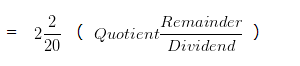Quotient = 2
Remainder = 2
Dividend = 20
We can use the following formula to convert Mixed Fraction into Improper fraction =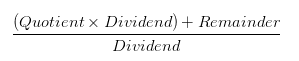So, given fraction can be written as

=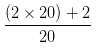= 42/20
Now, to convert a Fraction into Percentage, we need to multiply Fraction by 100 and add the sign of Percentage (%) to it
( Fraction x 100 ) %
= ( 42/20 x 100 ) %
= ( 42 x 5 ) %
= 210 %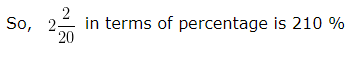Correct Answer – c) 210 %

Q.3) Explanation – Percentage Worksheets for Grade 5

In order to convert a Percentage into a Fraction, we Divide the Percentage by 100, and remove the sign of Percentage (%).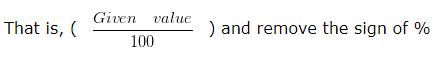we have,
75 % = 75/100
Now, to convert 75/100 to its lowest term, find the HCF of Numerator and Denominator
HCF of 75 and 100 is 25
Divide both 75 and 100 by their HCF i.e, 25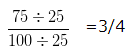Hence 75 % = 3/4

Q.4) Explanation – Percentage Worksheets for Grade 5

In order to convert given Percentage into into decimal, we have to perform the following steps:
Step 1 – Convert the Percentage into Fraction, by dividing the given Percentage by 100 and remove the sign of Percentage (%).
i.e, 25 % = 25/100
Step 2 – Convert the Fraction obtained at Step 1, into a Decimal form , by dividing numerator by Denominator
i.e, 25/100 = 0.25
Hence, Decimal form of 25 % is 0.25

Q.5) Explanation – Percentage Worksheets for Grade 5

In order to compare two quantities, both of them should be in same units. Here we have one unit in kg and other unit in g . So we would first convert both the quantities into same units
As we know that,
1 kg = 1000 g
Therefore,
6 kg = 6 x 1000 g
= 6000 g
To express 300 g as a Percentage of 6000 g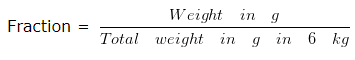Fraction =300/6000
Now, To convert Fraction into Percentage, we need to multiply the given Fraction by 100 and add the sign of Percentage (%) to the result.
So, (300/6000 x 100 ) %
On simplifying we get,
= 5 %
Hence, 300 g is 5 % of 6 kg .

Correct Answer – b) 5 %

Q.6) Explanation – Percentage Worksheets for Grade 5

Firstly we convert the Percentage into Fraction, by dividing the given percentage by 100, and then remove the sign of %
i.e, 12 % = 12/100
Since ‘of’ means multiplication
We would now, Multiply the Fraction with given quantity
or in other words 12/100 x 25
= 3
Hence, 12 % of 25 is 3

Q.7) Explanation – Percentage Worksheets for Grade 5

Percentage of Girls in the School = 70 %
We have to find Percentage of Boys in the School
Since, anything as a whole is cosidered as 100 %
So, Percentage of total number of Students in the School = 100 %
Percentage of Boys = Percentage of total number of Students – Percentage of Girls
= ( 100 – 70 ) %
Percentage of Boys = 30 %

Correct Answer – c) 30 %

Q.8) Explanation – Percentage Worksheets for Grade 5

Marks obtained by Divya = 30
Maximum marks = 50
We can write marks obtained by Radha as a Fraction of Maximum marks as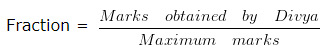Fraction = 30/50
Now, To convert Fraction into Percentage, we need to multiply the given Fraction by 100 and add the sign of Percentage (%) to the result.
So, ( 30/50 x 100 ) %
= 60 %
So, the Percentage of marks obtained by Divya = 60 %

Correct Answer – b) 60 %

Q.9) Explanation – Percentage Worksheets for Grade 5

Total number of children in a class = 88
Percentage of children who chose Sanskrit = 75 %
Number of children who chose Sanskrit = 75 % of 88
= ( 75/100 x 88 )
Number of children who chose Sanskrit = 66
Number of children who chose Hindi = Total number of children – Number of children who chose Sanskrit
= 88 – 66
Number of children who chose Hindi = 22

Correct Answer – c) Number of children who chose Sanskrit = 66 , Number of children who chose Hindi = 22

Q.10) Explanation – Percentage Worksheets for Grade 5

Total number of children in the class = 80
Number of children who went for picnic = 48
It can be written as,
Percentage of children who went for picnic =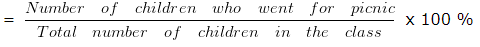Percentage of children who went for picnic = ( 48/80 x 100 ) %
Percentage of children who went for picnic = 60 %
Since, anything as a whole is considered as 100 %
So, Percentage of Total number of children = 100 %
Percentage of children who who did not go for the picnic = Percentage of Total number of children – Percentage of children who went for picnic
Percentage of children who who did not go for the picnic = ( 100 – 60 ) %
Percentage of children who who did not go for the picnic = 40 %

Correct Answer – a) Percentage of children who who did not go for the picnic = 40 % , Percentage of children who went for picnic = 60 %